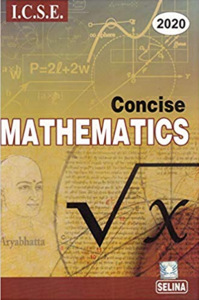Share

Selina solutions for Class 10 Mathematics chapter 10 - Arithmetic Progression

ICSE Concise Mathematics for Class 10 (2019-2020)

Chapters

Chapter 1: Value Added Tax

Chapter 2: Banking (Recurring Deposit Account)

Chapter 3: Shares and Dividends

Chapter 4: Linear Inequations (in one variable)

Chapter 5: Quadratic Equations

Chapter 6: Solving (simple) Problmes (Based on Quadratic Equations)

Chapter 7: Ratio and Proportion (Including Properties and Uses)

Chapter 8: Remainder And Factor Theorems

Chapter 9: Matrices

Chapter 10: Arithmetic Progression

Chapter 11: Geometric Progression

Chapter 12: Reflection (In x-axis, y-axis, x=a, y=a and the origin ; Invariant Points)

Chapter 13: Section and Mid-Point Formula

Chapter 14: Equation of a Line

Chapter 15: Similarity (With Applications to Maps and Models)

Chapter 16: Loci (Locus and its Constructions)

Chapter 17: Circles

Chapter 18: Tangents and Intersecting Chords

Chapter 19: Constructions (Circles)

Chapter 20: Cylinder, Cone and Sphere (Surface Area and Volume)

Chapter 21: Trigonometrical Identities (Including Trigonometrical Ratios of Complementary Angles and Use of Four Figure Trigonometrical Tables)

Chapter 22: Heights and Distances

Chapter 23: Graphical Representation (Histograms and Ogives)

Chapter 24: Measures of Central Tendency (Mean, Median, Quartiles and Mode)

Chapter 25: Probability

Selina ICSE Concise Mathematics Class 10 (2019-2020)Chapter 10: Arithmetic Progression

10.D10.E

Chapter 10: Arithmetic Progression Exercise 10.D solutions [Page 0]

The sum of three consecutive terms of an A.P. is 21 and the sum of their squares is 165. find these terms.

Chapter 10: Arithmetic Progression

10.D10.E

Selina ICSE Concise Mathematics Class 10 (2019-2020)Selina solutions for Class 10 Mathematics chapter 10 - Arithmetic Progression

Selina solutions for Class 10 Maths chapter 10 (Arithmetic Progression) include all questions with solution and detail explanation. This will clear students doubts about any question and improve application skills while preparing for board exams. The detailed, step-by-step solutions will help you understand the concepts better and clear your confusions, if any. Shaalaa.com has the CISCE ICSE Concise Mathematics for Class 10 (2019-2020) solutions in a manner that help students grasp basic concepts better and faster.

Further, we at Shaalaa.com are providing such solutions so that students can prepare for written exams. Selina textbook solutions can be a core help for self-study and acts as a perfect self-help guidance for students.

Concepts covered in Class 10 Mathematics chapter 10 Arithmetic Progression are Arithmetic Progression - Finding Their General Term, Arithmetic Progression - Finding Sum of Their First ‘N’ Terms., Simple Applications of Arithmetic Progression.

Using Selina Class 10 solutions Arithmetic Progression exercise by students are an easy way to prepare for the exams, as they involve solutions arranged chapter-wise also page wise. The questions involved in Selina Solutions are important questions that can be asked in the final exam. Maximum students of CISCE Class 10 prefer Selina Textbook Solutions to score more in exam.

Get the free view of chapter 10 Arithmetic Progression Class 10 extra questions for Maths and can use Shaalaa.com to keep it handy for your exam preparation

S